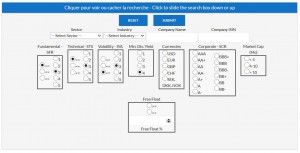# High Dividend Stocks – ENHigh Dividend Stocks

In this part, we screen our investment universe in order to keep/detect companies which respect some specific criteria:

1. We only want to keep companies with a gross dividend yield above 4%
2. We only want to keep companies which are not overvalued based on our valuation models -> Sigma Fundamental Rating (SFR) >= 3
3. We only want to keep companies which are ‘neutral’ or ‘positive’ on a technical basis approach (based on their trend and momentum) -> Sigma Technical Score (STS) >=3
4. We don’t want to keep companies with an higher price volatility than the average price volatility of our universe -> Sigma Volatility Level (SVL) <=3
5. Free Cash Flow Yield >= 1.25 * Dividend Yield (in order to secure the dividend)

Then, the result of this screening is split in 2 subgroups: European stocks and US stocks.Explanations on our different parameters:

• The gross dividend yield is computed by dividing the estimated gross dividend by the last weekly close of this specific stock. The result is expressed in percent.
• The Sigma Fundamental Rating (SFR) is a scoring scale we developed in order to make a link between the upside potential and the volatility level of a specific stock. So, a low volatility stock required less upside potential than a high volatility stock in order to justify a score of ‘4’ (attractive).

The scoring scale is determined as follow:

• SFR = ‘1’: the downside potential (based on our valuation models) is higher than -4 times the 50days price volatility expressed in percentage -> the stock is highly unattractive
• SFR = ’2’: the downside potential (based on our valuation models) is between -4 times and -2 times the 50days price volatility expressed in percentage -> the stock is unattractive
• SFR = ‘3’ : the estimated price change (based on our valuation models) is between -2 times and +2 times the 50days price volatility expressed in percentage -> the stock is neutral
• SFR = ‘4’ : the upside potential (based on our valuation models) is between +2 times and +4 times the 50days price volatility expressed in percentage -> the stock is attractive
• SFR = ‘5’ : the upside potential (based on our models) is greater than 4 times the 50days price volatility expressed in percentage -> the stock is highly attractive

• The Sigma Technical Score (STS) is a scoring scale we developed in order to score the trend and the momentum of a specific stock based on different time horizons (short term, medium term and long term).

The scoring scale is determined as follow:

• STS = ‘1’ : based on our algorithm dedicated to trend and momentum analysis, we consider the stock is highly unattractive
• STS = ‘2’ : based on our algorithm dedicated to trend and momentum analysis, we consider the stock is unattractive
• STS = ‘3’ : based on our algorithm dedicated to trend and momentum analysis, we consider the stock is neutral
• STS = ‘4’ : based on our algorithm dedicated to trend and momentum analysis, we consider the stock is attractive
• STS = ‘5’ : based on our algorithm dedicated to trend and momentum analysis, we consider the stock is highly attractive

• The Sigma Volatility Level (SVL) is a scoring scale we developed in order to estimate the volatility level of each stock within our investment universe.
• SVL = ‘1’ : the price volatility computed on the last 50 days is less than 50% of the average price volatility of our investment universe -> this stock was among the least volatile stock of our universe during the last 50 days.
• SVL = ‘2’ : the price volatility computed on the last 50 days is between 50% and 75% of the average price volatility of our investment universe -> this stock was less volatile than the rest of our investment universe.
• SVL = ‘3’ : the price volatility computed on the last 50 days is between 75% and 133% of the average price volatility of our investment universe -> this stock had a price volatility roughly in line with the price volatility of the rest of our universe.
• SVL = ‘4’ : the price volatility computed on the last 50 days is between 133% and 200% of the average price volatility of our investment universe -> this stock had a price volatility well above the average price volatility of the rest of our universe.
• SVL = ‘5’ : the price volatility computed on the last 50 days is greater than 2 times the average price volatility of our investment universe -> this stock was among the most volatile stock of our universe during the last 50 days.